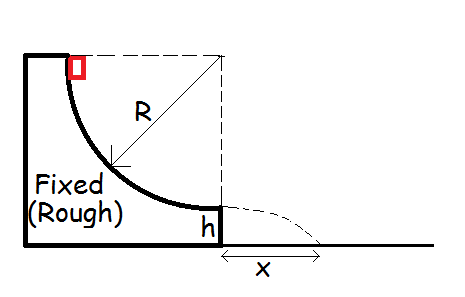# The Change Friction Makes In The Complexity Of A Problem.This problem has been inspired by a problem appearing in the JEE ADV -2013 (Paragraph questions 13-14). The problem was quite easy because work done by friction had been given and conservation of energy could be easily applied. I tried to find out how the work done by friction is evaluated.This try led to the formation of the following problem:

Consider a fixed wedge, whose part is a quarter circle of radius $R$ as shown. A small block of mass $m$ slides down the wedge and leaves horizontally from a height $h$ as shown.The coefficient of friction between wedge and block is $\mu_{k}$. Compute the value of $x$ in centimetres , where $x$ is the distance from bottom of wedge of the point where the block falls given the values below:

Details

• $R = 200 cm$

• $h = 105 cm$

• $\mu_{k} = 0.5$

• $m = 2g$

Round your answer to nearest integer

×

Problem Loading...

Note Loading...

Set Loading...